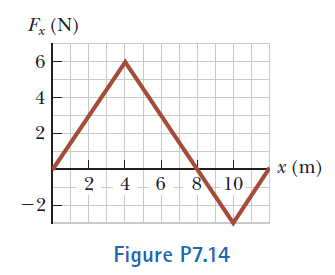# Problem: The force acting on a particle varies as shown in Figure P7.14. Find the work done by the force on the particle as it moves (a) from x = 0 to x = 8.00 m, (b) from x = 8.00 m to x = 10.0 m, and (c) from x = 0 to x = 10.0 m.

###### FREE Expert Solution

Work done by force over Δx is the area under the curve.

93% (449 ratings)###### Problem Details

The force acting on a particle varies as shown in Figure P7.14. Find the work done by the force on the particle as it moves (a) from x = 0 to x = 8.00 m, (b) from x = 8.00 m to x = 10.0 m, and (c) from x = 0 to x = 10.0 m.